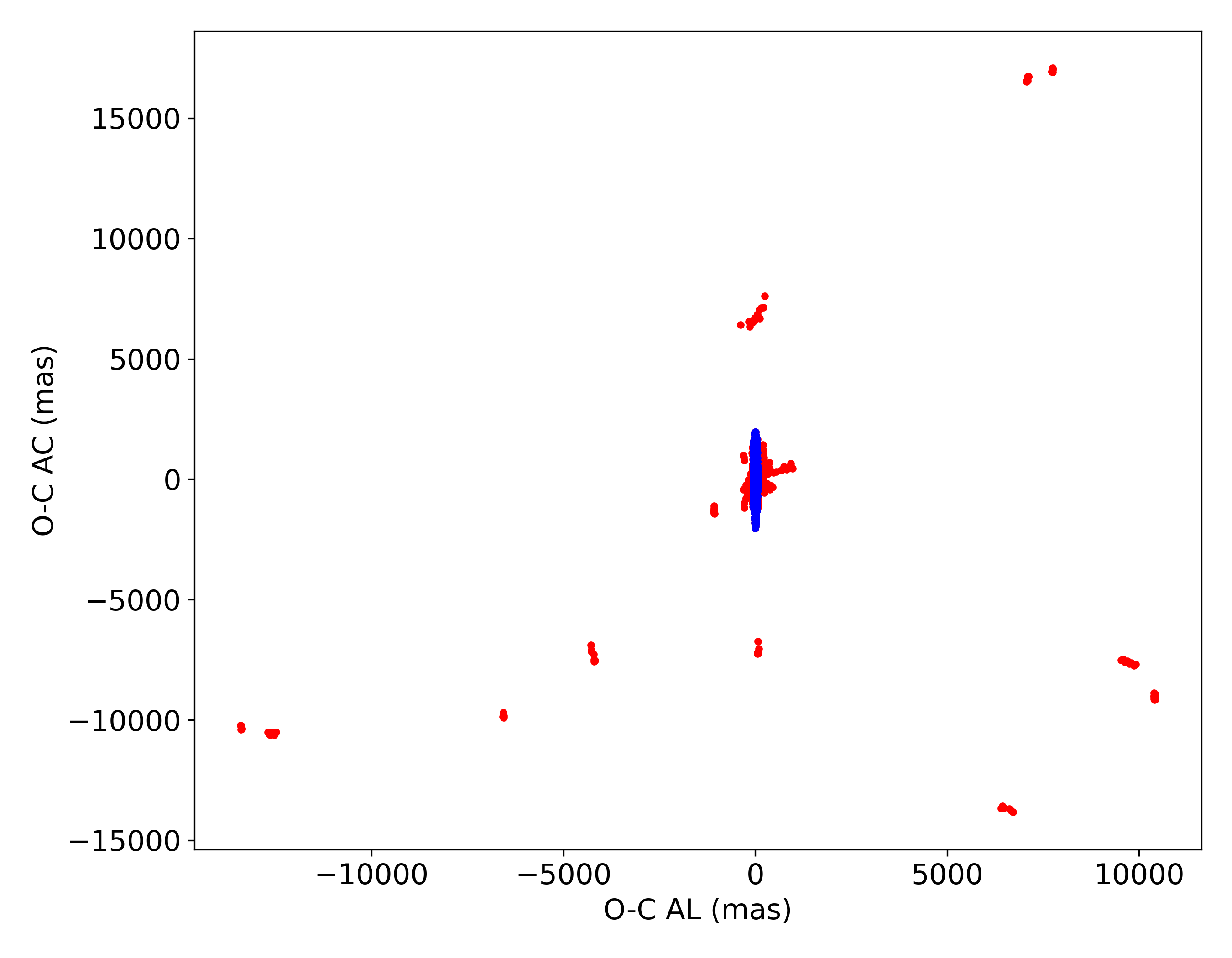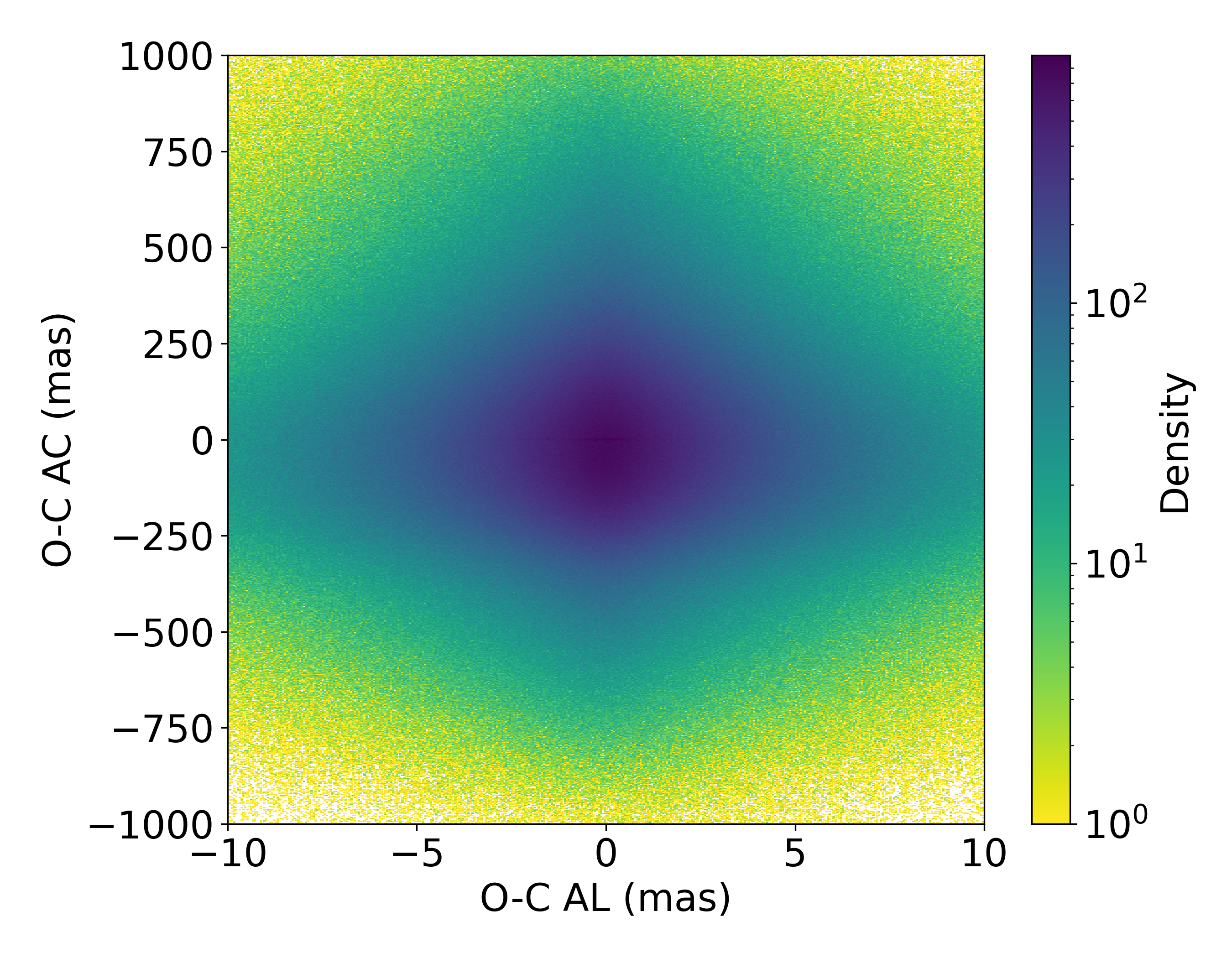# 14.8.2 Orbit determination results

We run an orbit determination fit for every known object. The process uses the known orbit as a starting point and then applies a non-linear weighted least-squares fit trying to minimise the residuals between the observations and the predictions done starting from the known orbit.

The weights used in the least-squares fit come directly from the published data and we make use of the full covariance matrices: systematic and random. To fully appreciate the quality of the data, the residuals need to be visualised in the ALong (AL) and ACross (AC) scan plane. How to obtain those residuals from the ones in right ascension and declination is already explained in Gaia Collaboration et al. (2018c).Figure 14.105: Post-fit residuals in the ALong scan - ACross plane (AL-AC) for all the observations of known objects. The plot shows how some observations have been rejected by the fit (red point).

Figure 14.105 shows the residuals in the $(AL-AC)$ plane for all the fitted observations. Red points correspond to rejected observations and the thick blue line in the centre of the figure contains all the accepted observations. Rejections can happen for different reasons, already explained in (Babusiaux et al. 2023). We want to point out that some of the very large residuals are related to mislinked objects. These misidentifications happen more often in high stellar density regions, where the density of observations is also low.

Figure 14.106 is a zoom in of what happens in the blue tick line of Figure 14.105. This density plot shows the residuals in the (AL-AC) plane for the observations selected by the fit procedure. Almost $95\%$ of post-fit residuals are concentrated in the $[-10,10]$ mas interval in AL and almost $79\%$ of observations have post-fit residuals in the $[-5,5]$ mas interval in AL.Figure 14.106: Density plot of the post-fit residuals in the ALong scan - ACross plane (AL-AC) in mas for the observations of known objects selected by the fit. The plot shows how that the highest density is concentrated in the [-5,5] mas interval in AL.

These numbers might seem low if compared to what found during the validation for Gaia DR2, where the $96\%$ of post-fit AL residuals where concentrated in the $[-5,5]$ mas interval.